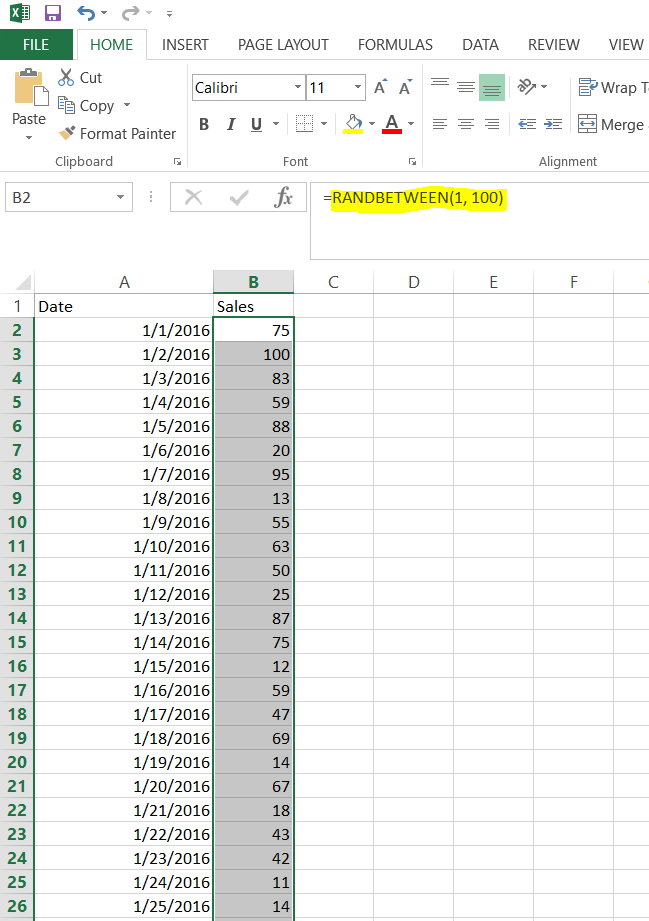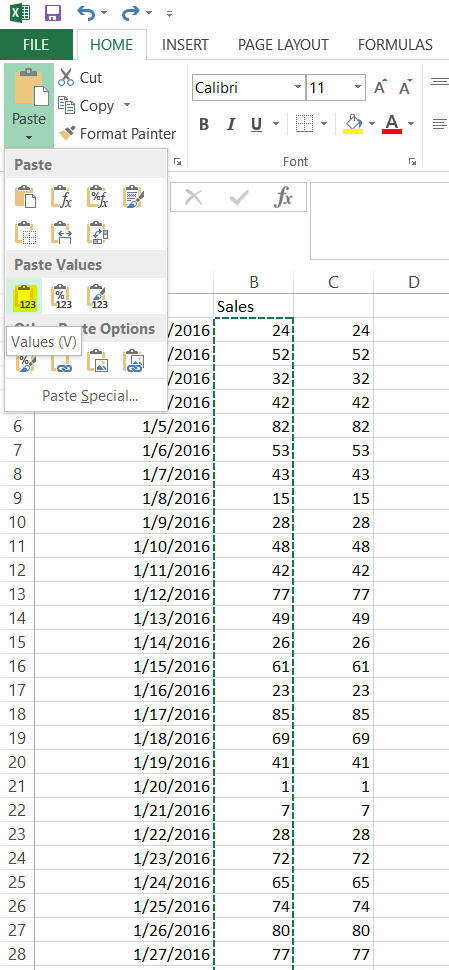# Excel Random Number Between

In this post we will generate random numbers for an Excel speadsheet.

In Excel, go to the cell or select the range where you would like the random number to occur.

Enter the formula:

`=RANDBETWEEN(lowernumber, uppernumber)`

For example, if we want to generate a number between 0 and 100, we would enter:

`=RANDBETWEEN(1, 100)`

Press Enter, or if you have selected multiple cells, press CTRL-ENTER. You will see the random numbers generated:If you need to remove the formula from the cell/cells, select the cells, copy and then paste the values into a new cell: